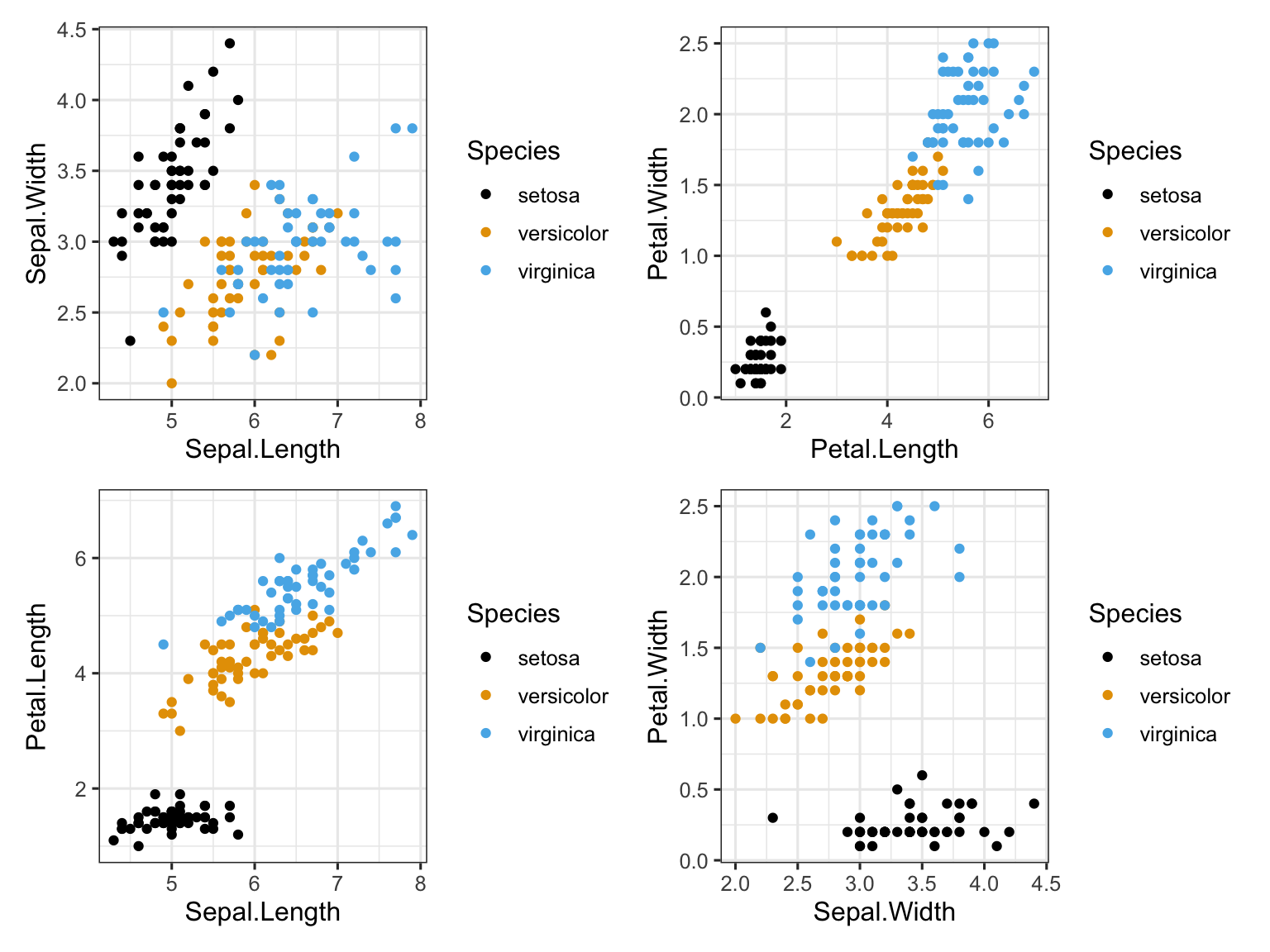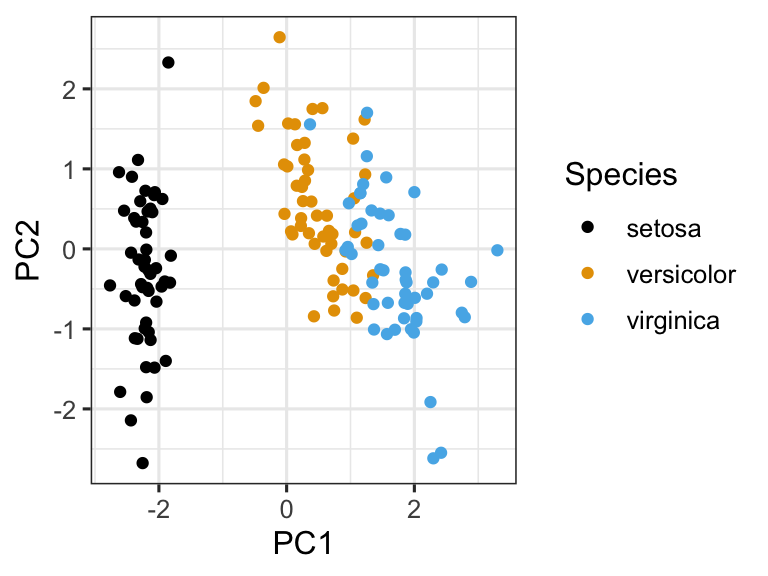## In-class worksheet 9

Feb 18, 2020

In this worksheet, we will use the libraries tidyverse, patchwork, grid, and ggthemes:

``````library(tidyverse)
theme_set(theme_bw(base_size=12)) # set default ggplot2 theme
library(patchwork) # required to arrange plots side-by-side
library(grid) # required to draw arrows
library(ggthemes) # for colorblind color scale``````

# 1. PCA of the iris data set

The `iris` dataset has four measurements per observational unit (iris plant):

``head(iris)``
``````##   Sepal.Length Sepal.Width Petal.Length Petal.Width Species
## 1          5.1         3.5          1.4         0.2  setosa
## 2          4.9         3.0          1.4         0.2  setosa
## 3          4.7         3.2          1.3         0.2  setosa
## 4          4.6         3.1          1.5         0.2  setosa
## 5          5.0         3.6          1.4         0.2  setosa
## 6          5.4         3.9          1.7         0.4  setosa``````

If we want to find out which characteristics are most distinguishing between iris plants, we have to make many individual plots and hope we can see distinguishing patterns:

``````p1 <- ggplot(iris, aes(x = Sepal.Length, y = Sepal.Width, color = Species)) +
geom_point() +
scale_color_colorblind()
p2 <- ggplot(iris, aes(x = Petal.Length, y = Petal.Width, color = Species)) +
geom_point() +
scale_color_colorblind()
p3 <- ggplot(iris, aes(x = Sepal.Length, y = Petal.Length, color = Species)) +
geom_point() +
scale_color_colorblind()
p4 <- ggplot(iris, aes(x = Sepal.Width, y = Petal.Width, color = Species)) +
geom_point() +
scale_color_colorblind()
p1 + p2 + p3 + p4 + plot_layout(ncol = 2) # arrange in a grid``````In this particular case, it seems that petal length and petal width are most distinct for the three species. Principal Components Analysis (PCA) allows us to systematically discover such patterns, and it works also when there are many more variables than just four.

The basic steps in PCA are to (i) prepare a data frame that holds only the numerical columns of interest, (ii) scale the data to 0 mean and unit variance, and (iii) do the PCA with the function `prcomp()`:

``````iris %>%
select(-Species) %>%   # remove Species column
scale() %>%            # scale to 0 mean and unit variance
prcomp() ->            # do PCA
pca                    # store result as `pca`

# now display the results from the PCA analysis
pca``````
``````## Standard deviations (1, .., p=4):
##  1.7083611 0.9560494 0.3830886 0.1439265
##
## Rotation (n x k) = (4 x 4):
##                     PC1         PC2        PC3        PC4
## Sepal.Length  0.5210659 -0.37741762  0.7195664  0.2612863
## Sepal.Width  -0.2693474 -0.92329566 -0.2443818 -0.1235096
## Petal.Length  0.5804131 -0.02449161 -0.1421264 -0.8014492
## Petal.Width   0.5648565 -0.06694199 -0.6342727  0.5235971``````

The main results from PCA are the standard deviations and the rotation matrix. We will talk about them below. First, however, let’s plot the data in the principal components. Specifically, we will plot PC2 vs. PC1. The rotated data are available as `pca\$x`:

``head(pca\$x)``
``````##            PC1        PC2         PC3          PC4
## [1,] -2.257141 -0.4784238  0.12727962  0.024087508
## [2,] -2.074013  0.6718827  0.23382552  0.102662845
## [3,] -2.356335  0.3407664 -0.04405390  0.028282305
## [4,] -2.291707  0.5953999 -0.09098530 -0.065735340
## [5,] -2.381863 -0.6446757 -0.01568565 -0.035802870
## [6,] -2.068701 -1.4842053 -0.02687825  0.006586116``````

As we can see, these data don’t tell us to which species which observation belongs. We have to add the species information back in:

``````# add species information back into PCA data
pca_data <- data.frame(pca\$x, Species = iris\$Species)
``````##         PC1        PC2         PC3          PC4 Species
## 1 -2.257141 -0.4784238  0.12727962  0.024087508  setosa
## 2 -2.074013  0.6718827  0.23382552  0.102662845  setosa
## 3 -2.356335  0.3407664 -0.04405390  0.028282305  setosa
## 4 -2.291707  0.5953999 -0.09098530 -0.065735340  setosa
## 5 -2.381863 -0.6446757 -0.01568565 -0.035802870  setosa
## 6 -2.068701 -1.4842053 -0.02687825  0.006586116  setosa``````

Now we can plot as usual:

``````ggplot(pca_data, aes(x = PC1, y = PC2, color = Species)) +
geom_point() +
scale_color_colorblind()``````In the PC2 vs PC1 plot, versicolor and virginica are much better separated.

Next, let’s look at the rotation matrx:

``pca\$rotation``
``````##                     PC1         PC2        PC3        PC4
## Sepal.Length  0.5210659 -0.37741762  0.7195664  0.2612863
## Sepal.Width  -0.2693474 -0.92329566 -0.2443818 -0.1235096
## Petal.Length  0.5804131 -0.02449161 -0.1421264 -0.8014492
## Petal.Width   0.5648565 -0.06694199 -0.6342727  0.5235971``````

It tells us how much each variable contributes to each principal component. For example, `Sepal.Width` contributes little to PC1 but makes up much of PC2. Often it is helpful to plot the rotation matrix as arrows. This can be done as follows:

``````# capture the rotation matrix in a data frame
rotation_data <- data.frame(
pca\$rotation,
variable = row.names(pca\$rotation)
)

# define a pleasing arrow style
arrow_style <- arrow(
length = unit(0.05, "inches"),
type = "closed"
)

# now plot, using geom_segment() for arrows and geom_text() for labels
ggplot(rotation_data) +
geom_segment(aes(xend = PC1, yend = PC2), x = 0, y = 0, arrow = arrow_style) +
geom_text(aes(x = PC1, y = PC2, label = variable), hjust = 0, size = 3, color = "red") +
xlim(-1., 1.25) +
ylim(-1., 1.) +
coord_fixed() # fix aspect ratio to 1:1``````